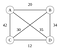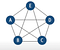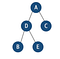# Travelling Salesman Problem — The Optimization Challenge To Rule Them All.

“Given a list of cities and the distances between each pair of cities, what is the shortest possible route that visits each city exactly once and returns to the origin city?”

This seemingly benign question has had a vast influence in its purest formulation within the areas of theoretical computer science, planning and logistics, and the manufacture of microchips. It is known as the Travelling Salesman Problem. Modified versions of this problem have even had applications in disparate areas, from DNA sequencing to astronomy. In the following post I will sketch a brief outline the problem, illuminate the computational complexity and how it is categorized in the realm of computer science, and finally provide a couple of solutions which have been used in recent decades. I hope to show how the concepts of computer science, applied mathematics, and algorithm design can be utilized to solve everyday problems.

# BeginningsWeighted Symmetric TSP

The origins of the Traveling Salesman Problem, or TSP, can be traced back to the early 19th century, but it was first formalized mathematically by an individual named Merrill M. Flood in 1930, who was attempting to solve a school bus routing problem. Later taken up by Hassler Whitney at Princeton under the moniker of the “48 states problem”, in which the shortest route was considered for visiting all of the contiguous 48 United States, it was finally given the current “TSP” phrase in a RAND Corporation report dating from 1949.

The RAND corporation played a key role in popularizing the issue, at least in scientific circles, and a team there wrote what would become a seminal paper on the subject, which introduced the ‘cutting plane’ method to solve an instance of traveling to 49 cities to optimality, in the 1960s. However, later TSP formulations improved on the problem by offering “near optimal” solutions which could be computed algorithmically, and were able to cut down on the number of routes significantly.

Algorithmic approaches would continue to improve upon the problem, first by modifying cutting plane scenario, and later by “branch and bound” methods. Currently, the largest solved instance of TSP involves 85,900 nodes, which was used in a microchip layout design; however, other formulations have been applied to near optimality which involve millions of cities, or nodes. A slightly improved approximation algorithm was developed in 2020.

## The Challenge

Now we will look at a method for solving a TSP instance, which will exemplify the scope of the problem. So what makes the Traveling Salesman Problem so computationally difficult? Well, even a cursory observation of the problem can reveal its complexity. TSP problems often involve graph and tree data structures; in our formulation, we can begin by using a weighted, undirected, complete graph. The graph is weighted, meaning the edges/routes represent different values to account for the varying distances between the cities, and direction travelled is the same value, so it is considered undirected. Finally, a graph is considered complete when each vertex of the graph is connected to every other vertex via routes. We are also going to be looking at an approximation example for the sake of simplicity, although more exacting formulations have been devised.

The approach used in our example will be through what is called the Hamiltonian cycle, which requires that each vertex, or city, shall be visited exactly once, and that the route must end at the starting point. Using our graph, we will look at the MST-DFS method, which requires finding the Minimum Spanning Tree of the graph, as well as a Depth First Search traversal of the tree.Complete graph

Beginning with the complete graph, we first designate a root vertex (A), and find the shortest edge that goes to an unvisited vertex (E), and keep adding the cheapest edge which goes to another unvisited vertex, until every vertex is visited in the tree. Hence we have a minimum spanning tree, which is the cheapest way of getting from the root vertex to every other vertex.Graph transformed into a minimum spanning tree

The next step is the depth first search traversal. Starting at root node (A), we descend down the tree to its deepest point (B), and then work our way back up, then descend down the other branch of the tree (E), return back up to the root, and finally descend down the last branch of our small example (C). This traversal may be written as follows: A →D →B →D →E →D →A →C →A.

The final step in this method is simply to remove the duplicate vertices traversed during the DFS phase. This leaves: A →D →B →E →C →A, and thus completes the Hamiltonian cycle. Using this approach, the MST tour, may be greater than or equal to the most optimal solution, but is guaranteed to be less than or equal to two MSTs (the result of covering each edge of the minimum spanning tree twice). The optimal solution would be a single traversal of the MST, and the worst case is twice that optimum (2 MST or 2 Opt.), so this is called a 2-Approximation algorithm. Other solutions follow this same nomenclature, such that a method known as Christofides’ algorithm is considered a 3/2 Approximation algorithm.

# Classification & Computation

As shown in the simple example above, any approach to solving the Travelling Salesman Problem is predicated on how to determine the preference of paths given many possibilities. As the number of nodes grows larger, the number of potential solutions goes up exponentially, and thus the computation required to compare each solution to find the optimum one. The number of possibilities for a TSP problem may be calculated as: (n — 1) ! / 2, where ! is factorial notation. The factorial of any integer is the integer multiplied by itself minus one, then that product times itself minus two, and so on until one is reached. Thus, 4! translates to (4*3*2*1 = 24).

Solving for the number of possibilities seems straightforward then, right? Well, suppose we would like to solve a TSP instance which has 30 cities. This would yield 30!/2 solutions which need to be compared. Today’s computers can perform an incredible number of calculations per second. Yet even if we used a computer which can perform 10⁶ calculations in one second, using our formula — 30! / (2*10⁶) seconds would reduce down to… 6.3* 10¹³… YEARS! This is probably longer than we would like to wait to determine the optimal solution. Therefore it is essentially impossible to compare this many possible routes for even a relatively small number of cities such as 30.

TSPs are classified as NP-Hard problems for this reason. NP stands for non-deterministic polynomial-time, and running an exact algorithm would be O(n!) time complexity. Amazingly, some exact solutions for TSPs have been computed, including one which in 2004 found the optimal route for visiting 24,978 locations in Sweden.

Therefore, much more practical approaches to solving TSPs are called heuristic or approximation methods. They do not guarantee an absolute optimum route, but are able to come within 99% certainty. Approximation algorithms include the MST-DFS approach covered above, as well as countless others which take a range of creative approaches.

# Why Bother?

The Travelling Salesman Problem is an optimization challenge that acts like a key to solving other mathematical problems that are thought to be hard; it has been proven that a quick travelling salesman algorithm, if one exists, could be converted into quick algorithms for many other difficult tasks, such as factoring large numbers. Since many cryptographic schemes rely on the difficulty of factoring integers to protect their data, this would allow access to private data like personal correspondence, bank accounts and, possibly, government secrets.

For modified applications of TSP, the concept city may represent customers, soldering points, or DNA fragments, and the concept distance represents travelling times or cost, or a similarity measure between DNA fragments. The TSP also appears in astronomy, as astronomers observing many sources will want to minimize the time spent moving the telescope between the sources; in such problems, the TSP can be imbedded inside an optimal control problem.

Thus the potential applications are immense, making TSP a benchmark for many optimization methods. The most straightforward application is in logistics: companies such as Amazon rely on computations like TSP as well as a related algorithm called the Vehicle Routing Problem to solve routing issues via automation in order to deliver millions of packages daily. It is inevitable that this problem will continually evolve and adapt to changing organizational structures in society and the commensurate technology used therein. I hope this has been a useful introduction into one of the most interesting problems in the history of computer science, and I implore the reader to explore the many fascinating approaches and attempts to solve this most elusive of programming challenges. Thank you for reading!